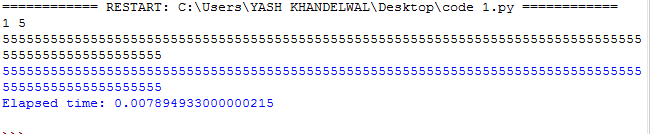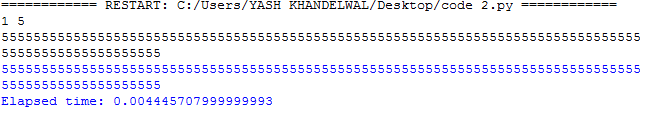# Fast input / output for competitive programming in Python

Python sys.stdin.readline() and sys.stdout.write() functions: Here, we are going to learn how to take fast input in python for competitive programming?
Submitted by Yash Khandelwal, on April 04, 2019

In competitive programming it is very important to make the program time efficient. And to make that one major problem is input and output. As input and output of the program occupy more time. So, because of that, the efficiency of code get decreased.

There are some of the specific functions that can be used for fast input and output.

Python provides two file objects "stdin" and "stdout" while are a part of "sys" module, we can use readline() method of "stdin" for input and write() function of "stdout" for output.

It is used to take the input, it takes the input as a string by default and if you want to take input as an integer then use the eval() or int() functions. If you want to take space separated inputs in a single line then use split().

Example:

```    stdin.readline(eval(input().split()))
```

instead of eval() you can also use int(). If you do not use any of these functions, input will be string by default

2) stdout.write()

It gives the output in string type. And if you want the multiple outputs in a single statement, use '+' in the between the two.

Example:

```    stdout.write('p', + ,'A', +, 'C')
```

Syntaxes:

```    stdin.readline()    # for input
stdout.write()      # for output
```

To use the fast input output functions, you need to import the files which contain them i.e. "sys module".

Example 1: Python code for simple input, output

```# python program without fast I/O
from time import perf_counter

#integer input from user, 2 input in single line
n,m = map(int,input().split())

t1_start = perf_counter()
for i in range(n):
t=int(input()) # user gave input n times
if t%m == 0:
print(t) #print the output if condition satisfied

t1_stop = perf_counter()# Stop the stopwatch/counter

print("Elapsed time:", t1_stop-t1_start) # Report results
```

OutputExample 2: Python code for fast input, output

```# python program with fast I/O

#module contain stdin ,stdout
from sys import stdin, stdout
from time import perf_counter

#integer input from user ,2 input in single line
n,m=map(int,input().split())

t1_start = perf_counter()

for i in range(n):
t=int(stdin.readline()) # input using fast i/p method
if t%m == 0:
stdout.write(str(t)+'\n') #input using fast o/p method

t1_stop = perf_counter()# Stop the stopwatch

print("Elapsed time:", t1_stop-t1_start) # Report results

```

OutputIt takes 0.004445707999999993 sec time to execute from input to output

Note: As you can see in the above code, that time taken by fast input method to run a 100 digit line is half the time taken in simple Input/Output.

Preparation